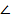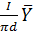# A single-phase half-controlled rectifier is driving a separately excited dc motor.The dc motor has a back emf constant of 0.5 V/rpm.The armature current is 5 A without any ripple.The armature resistance is 2Ω,The converter is working from a 230 V,single phase ac source with a firing angle of 30o.Under this operating condition,the speed of the motor will be

1.  339 rpm

2.  359 rpm

3.  366 rpm

4.  386 rpm

4

366 rpm

Explanation :
No Explanation available for this question

# A variable speed drive rated for 1500 rpm,40 Nm is reversing under no load.figure shows the reversing torque and the speed during the transient.The moment of inertia of the drive is

1.  0.048 kg m2

2.  0.064 kg m2

3.  0.096 kg m2

4.  0.128 kg m2

4

0.096 kg m2

Explanation :
No Explanation available for this question

# Figure shows the waveform of the current passing through an inductor of resistance 1Ω and inductance  2H.The energy absorbed by the inductor in the first four seconds is

1.  144 J

2.  98 J

3.  132 J

4.  168 J

4

144 J

Explanation :
No Explanation available for this question

# A segment of a circuit is shown in he figure Q2.VR=5V,VC=4 sin 2t.The voltage VL is given by

1.  3-8 cos 2t

2.  32 sin 2t

3.  16 sin 2t

4.  16 cos 2t

4

32 sin 2t

Explanation :
No Explanation available for this question

# In the figure.Q3.Z1=10 -60O,A2=10 60O,Z3=50 53.13O.The venn impedance seen from X-Y is

1.  56.6 45o

2.  60 30o

3.  70 30o

4.  34.4 65o

4

56.645o

Explanation :
No Explanation available for this question

# Two conductors are carrying forward and return current of +I and –I as shown in figure.The magnetic field intensity at point P is

1.

2.

3.

4.

4Explanation :
No Explanation available for this question

# Two infinite strips of width W m in x direction as shown in the figure are carrying forward and return currents +I and –I in the z direction.The strips separated by a distance of xm.The inductance per unit lengt of the configuration is measured to be L H/m.if the distance of separation between the strips is now reduced to x/2 m,the inductance per unit length of the configuration is

1.  2L H/m

2.  L/4 H/m

3.  L/2 H/m

4.  4L H/m

4

L/2 H/m

Explanation :
No Explanation available for this question

# A single phase transformer has a maximum efficiency of 90% at full load and unity power factor.Efficiency at half load at the same power factor is

1.  86.7%

2.  88.26%

3.  88.9%

4.  87.8%

4

87.8\%

Explanation :
No Explanation available for this question

# A stand-alone engine driven synchronous generator is feeding a partly inductive load.A capacitor is now connected across the load to completely nullify the inductive current.For  this operating condition

1.  the field current and fuel input have to be reduced

2.   the field current and fuel input have to be increased

3.   the field current has to be increased and fuel input left unaltered

4.   the field current has to be reduced and fuel input left unaltered

4

the field current has to be reduced and fuel input left unaltered

Explanation :
No Explanation available for this question

# Curves X and Y in the figure denotes open circuit and full-load zero power factor(zpf) characteristics of a synschronous generator.Q is a point on the zpf characteristics at 1.0p.u voltage.The vertical distance PQ in figure gives the voltage drop across

1.  synchronous reactance

2.  Magnetizing reactance

3.  potier reactance

4.  leakage reactance

4